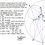Explanation for an easy(not for me) physics question

I don't think this would've been a problem on Brilliant since I found it in a physics workbook, the problem is: A boy stands on a 50-m-high bridge with his GI Joe. The action figure has a real parachute. The boy drops Joe from the bridge, but tragically the chute fails to deploy. If the action figure has a mass of 100 g and it never reaches terminal velocity, how fast will Joe be going when he strikes the river?

After reading this question, at first I was confused about why the mass of the object is given. Since the object experiences a constant gravitational pull from earth regardless of its mass, couldn't we just use the formula $50=\frac{at^2}{2}$ to find the time then multiply it by a(or 9.8)? Surprisingly, the answer given in the back of the book used the conservation of energy(with a different answer also),which I would never have thought of.Why??? Do I use conservation of energy in a problem whenever the mass of an object is given?(doesn't make any sense...)

Second question which is not about the problem above: It seems like a lot of ideas in physics are really abstract. I managed to understand and visualize force and work, but not momentum and power yet. How do you guys overcome this problem? I would like to know.

Sorry for my ignorance :(. Thank you.Note by Xuming Liang
6 years, 3 months ago

This discussion board is a place to discuss our Daily Challenges and the math and science related to those challenges. Explanations are more than just a solution — they should explain the steps and thinking strategies that you used to obtain the solution. Comments should further the discussion of math and science.

When posting on Brilliant:

• Use the emojis to react to an explanation, whether you're congratulating a job well done , or just really confused .
• Ask specific questions about the challenge or the steps in somebody's explanation. Well-posed questions can add a lot to the discussion, but posting "I don't understand!" doesn't help anyone.
• Try to contribute something new to the discussion, whether it is an extension, generalization or other idea related to the challenge.
• Stay on topic — we're all here to learn more about math and science, not to hear about your favorite get-rich-quick scheme or current world events.

MarkdownAppears as
*italics* or _italics_ italics
**bold** or __bold__ bold

- bulleted
- list

• bulleted
• list

1. numbered
2. list

1. numbered
2. list
Note: you must add a full line of space before and after lists for them to show up correctly
paragraph 1

paragraph 2

paragraph 1

paragraph 2

> This is a quote
This is a quote
# I indented these lines
# 4 spaces, and now they show
# up as a code block.

print "hello world"
# I indented these lines
# 4 spaces, and now they show
# up as a code block.

print "hello world"
MathAppears as
Remember to wrap math in $$...$$ or $...$ to ensure proper formatting.
2 \times 3 $2 \times 3$
2^{34} $2^{34}$
a_{i-1} $a_{i-1}$
\frac{2}{3} $\frac{2}{3}$
\sqrt{2} $\sqrt{2}$
\sum_{i=1}^3 $\sum_{i=1}^3$
\sin \theta $\sin \theta$
\boxed{123} $\boxed{123}$

Sort by:

There's nothing wrong with your approach. A slightly shorter way is this:

$v=\sqrt{2gh}$

where, $v$ is Joe's final velocity, $g$ is the gravitational acceleration and $h$ is the height Joe is falling from.

If we plug in the values we get $v=\sqrt{2\times9.8\times50} ms^{-1}$ or approximately $31.3 ms^{-1}$.

We don't need the mass to figure this out. We can use the law of conservation of energy, but it'll give us the same result. The mass terms will cancel out. I don't know why your book has a different answer.

- 6 years, 3 months ago

try to read paul g,hewitt concepts of physics it 'll help and comin 2 the 1st one u must get the same answer in both ways

- 6 years, 3 months ago